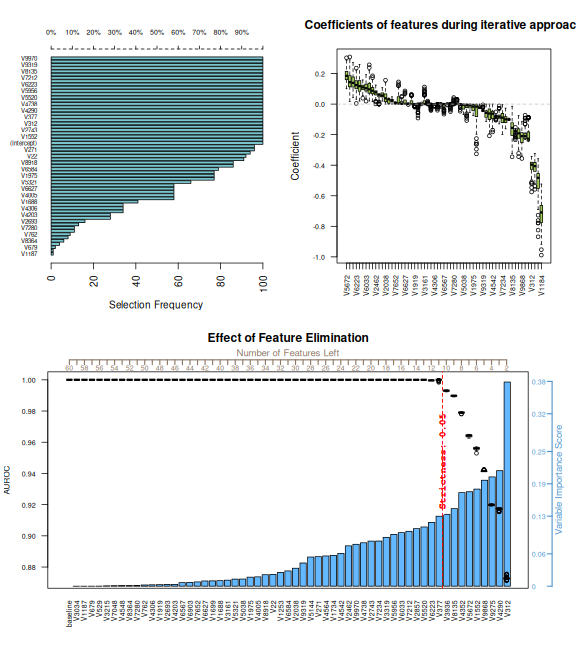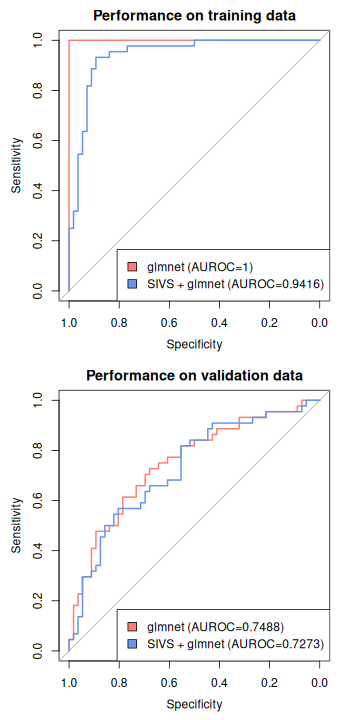# Tutorial of basic Usage

## Description

Stable Iterative Variable Selection (SIVS) is a feature selection method that wraps machine learning methods with internal feature selection (e.g shrinkage methods such as LASSO). In this vignette we demonstrate simple usage of this method. We would like to encourage users to always stay critical of the results they get and not use SIVS as a blackbox method.

For the purpose of this simple tutorial, we use Arcene Data Set which is a sample dataset to demonstrate feature selection methods via a binary classification. As the webpage states:

ARCENE’s task is to distinguish cancer versus normal patterns from mass-spectrometric data.

``````train_x <- read.csv(file = "https://archive.ics.uci.edu/ml/machine-learning-databases/arcene/ARCENE/arcene_train.data", header = F, sep = " ", strip.white = T, blank.lines.skip = T, stringsAsFactors = F)
train_y <- read.csv(file = "https://archive.ics.uci.edu/ml/machine-learning-databases/arcene/ARCENE/arcene_train.labels", header = F, sep = " ", strip.white = T, blank.lines.skip = T, stringsAsFactors = F)

validation_x <- read.csv(file = "https://archive.ics.uci.edu/ml/machine-learning-databases/arcene/ARCENE/arcene_valid.data", header = F, sep = " ", strip.white = T, blank.lines.skip = T, stringsAsFactors = F)
validation_y <- read.csv(file = "https://archive.ics.uci.edu/ml/machine-learning-databases/arcene/arcene_valid.labels", header = F, sep = " ", strip.white = T, blank.lines.skip = T, stringsAsFactors = F)

train_y <- train_y[, 1]
validation_y <- validation_y[, 1]
``````

## Explore, Pre-process, and clean data

Check for possible missing values in the data:

``````library("varhandle")

knitr::kable(varhandle::inspect.na(d = train_x, barplot = F))
``````
column_index column_name number_of_NAs ratio_of_NA
10001 V10001 100 1
``````knitr::kable(varhandle::inspect.na(d = validation_x, barplot = F))
``````
column_index column_name number_of_NAs ratio_of_NA
10001 V10001 100 1

There is a column (V10001) that has 100% `NA` in each of the two datasets (probably because the data is not CSV and some white-space issues). Let’s remove that and move on:

``````train_x <- train_x[, -10001]
validation_x <- validation_x[, -10001]
``````

Now let’s have a better understanding of what variables we are dealing with in this R session:

``````knitr::kable(varhandle::var.info(regex = "_[xy]\$"))
``````
name class size detail
train_x data.frame 5 Mb dimension: 100, 10000
validation_x data.frame 5 Mb dimension: 100, 10000
train_y integer 448 bytes length: 100
validation_y integer 448 bytes length: 100

## Using SIVS

Now we can run SIVS to get the most important features. Note that for the sake of making the vignette, the progressbar is set to FALSE and the verbosity has set to none so that the function does not print its progress. It’s worth noting that due to the nature of this feature selection method, running a high-dimensional data in SIVS would take time, therefore, the more CPU core you allocate to it, the faster it will do the job. The following would take about 19 minutes with 2 CPU cores (`parallel.cores = 2`) and it will be much faster if you use `parallel.cores = "grace"` which will use all CPU cores except 1.

``````library("sivs")

sivs_obj <- sivs::sivs(x = train_x,           # it should be a matrix or dataframe with features as columns and samples as rows
y = factor(train_y),
verbose = "none",      # This is for demonstration, you leave the verbose to be "general"
progressbar = FALSE)   # This is for demonstration, you leave the progressbar on
``````

By providing factor with 2 levels, we are implying to use binomial. Alternatively, you can define `family = "binomial"` and it will be passed to internal method (which the default is glmnet).

Having the SIVS object, we can plot the object to have better understanding of what it has found:

``````layout(mat = matrix(c(1,2,
3,3),
nrow = 2,
byrow = T))
{
plot(sivs_obj)
layout(1)
}
``````The top-left plot shows the how many times each feature was selected in the iterative step. The top-right plot shows the coefficient distribution of each of the features selected in the iterative step. This plot is sorted based on the median of the coefficient of each feature. Lastly, the bottom plot shows the result of recursive feature elimination (rfe) step. Each blue bar shows the importance of that feature, whereas each boxplot shows the distribution of areas under the receiver operating characteristic curves (AUROC) after removal of that feature. The more we move towards left-hand side of the plot the more features we have removed and as expected, the AUROCs start decreasing. The read vertical line is the suggested cutoffs based on the `sivs::suggest()` function when provided by the `sivs_obj`. Note that In this example due to the data we see only the 0.05 suggested threshold, but in datasets with more difficult setup that have more of less-important features, we would also see another suggestion as a vertical red line for 0.01 cutoff. These cutoffs are suggestions and users are encourage to treat them as such. These two cutoffs are pre-defined in the function as loose cutoffs and we encourage users to investigate the suggested features and make the cutoff more strict is possible according to their data and setup and the question they are trying to answer.

Finally, we can extract the list of suggested features by SIVS. Considering that we cannot see 0.01 line in the bottom plot of the figure above and the 0.05 is where the boxplots start dropping, let’s choose a stricter threshold. The strictness can be between 0 (very loose) to 1 (very strict). Here for the sake of the example, we use 0.5 as it is the exact middle of the strictness range:

``````sivs::suggest(sivs_obj, strictness = 0.5)
#>  "V1184" "V698"  "V312"  "V4290" "V9275"
``````

Ultimately, one can compare the performance of a machine learning method with and without SIVS. In the following example we use `glmnet` package as in this vignette it was used by SIVS as the internal method.

``````library("glmnet")
library("pROC")
#> Type 'citation("pROC")' for a citation.
#>
#> Attaching package: 'pROC'
#> The following objects are masked from 'package:stats':
#>
#>     cov, smooth, var

# build a model without SIVS
set.seed(12345)
glmnet_model <- glmnet::cv.glmnet(x = data.matrix(train_x),
y = factor(train_y),
family = "binomial")

# build a model with SIVS
set.seed(12345)
sivs_glmnet_model <- glmnet::cv.glmnet(x = data.matrix(train_x[, sivs::suggest(sivs_obj, strictness = 0.5)]),
y = factor(train_y),
family = "binomial")

# predict both training set and validation sets
glmnet_train_pred <- predict(object = glmnet_model,
newx = data.matrix(train_x),
s = "lambda.min",
type = "response")
glmnet_validation_pred <- predict(object = glmnet_model,
newx = data.matrix(validation_x),
s = "lambda.min",
type = "response")

sivs_glmnet_train_pred <- predict(object = sivs_glmnet_model,
newx = data.matrix(train_x[, sivs::suggest(sivs_obj, strictness = 0.5)]),
s = "lambda.min",
type = "response")
sivs_glmnet_validation_pred <- predict(object = sivs_glmnet_model,
newx = data.matrix(validation_x[, sivs::suggest(sivs_obj, strictness = 0.5)]),
s = "lambda.min",
type = "response")

glmnet_train_roc <- pROC::roc(response = factor(train_y),
predictor = as.numeric(glmnet_train_pred))
#> Setting levels: control = -1, case = 1
#> Setting direction: controls < cases
glmnet_validation_roc <- pROC::roc(response = factor(validation_y),
predictor = as.numeric(glmnet_validation_pred))
#> Setting levels: control = -1, case = 1
#> Setting direction: controls < cases

sivs_glmnet_train_roc <- pROC::roc(response = factor(train_y),
predictor = as.numeric(sivs_glmnet_train_pred))
#> Setting levels: control = -1, case = 1
#> Setting direction: controls < cases
sivs_glmnet_validation_roc <- pROC::roc(response = factor(validation_y),
predictor = as.numeric(sivs_glmnet_validation_pred))
#> Setting levels: control = -1, case = 1
#> Setting direction: controls < cases

layout(mat = matrix(1:2, nrow = 2))
{
plot(glmnet_train_roc, col = "salmon", main = "Performance on training data")
plot(sivs_glmnet_train_roc, col = "cornflowerblue", add = T)
legend("bottomright",
fill = c("salmon", "cornflowerblue"),
legend = c(paste0("glmnet (AUROC=",
round(pROC::auc(glmnet_train_roc),
digits = 4),
")"),
paste0("SIVS + glmnet (AUROC=",
round(pROC::auc(sivs_glmnet_train_roc),
digits = 4),
")")))

plot(glmnet_validation_roc, col = "salmon", main = "Performance on validation data")
plot(sivs_glmnet_validation_roc, col = "cornflowerblue", add = T)
legend("bottomright",
fill = c("salmon", "cornflowerblue"),
legend = c(paste0("glmnet (AUROC=",
round(pROC::auc(glmnet_validation_roc),
digits = 4),
")"),
paste0("SIVS + glmnet (AUROC=",
round(pROC::auc(sivs_glmnet_validation_roc),
digits = 4),
")")))
layout(1)
}
``````As we can see, the model build without SIVS has AUROC of 74.88% on validation set where as the model built based on the features selected by SIVS has AUROC of 72.73%. Now let’s compare the number of selected features:

``````# extract the coefficients
sum(coef(glmnet_model) != 0)
#>  29
sum(coef(sivs_glmnet_model) != 0)
#>  5
``````

This shows that without SIVS we would have selected 29 features, but with SIVS we have reduced it to just 5.